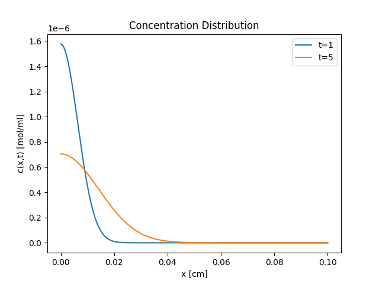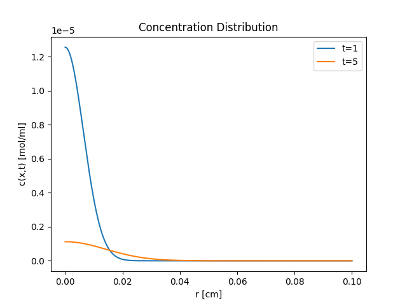# Team:TokyoTech/Model

Modeling

To confirm our bacteria have effects, we estimated the extent to which cinnamaldehyde diffuses when lactic acid bacteria synthesize it. From these results, we were able to determine the effective distance of lactic acid bacteria that actually exhibit antibacterial activity. We have extended the famous Fick's diffusion equation to three dimensions and predicted it by substituting the concentration of cinnamaldehyde that shows antimicrobial activity as determined experimentally.

Fick's diffusion equation in one dimension is as follows

$J\ \propto\ -\frac{\partial\ c}{\partial\ x}\ \rightarrow\ J\ =\ -D\frac{\partial\ c}{\partial\ x}$$\frac{\partial}{\partial\ t}\ \int_{x0}^{x1}\ c(x,\ t)dx\ =\ J(x0,\ t)\ -\ J(x1,\ t)$$\frac{\partial\ c}{\partial\ t}\ =\ D(\frac{\partial}{\partial\ x})^2\ c$ Since the diffusion coefficient D of cinnamaldehyde could not be determined this time, the known value of 0.00002 [㎠/s] for acetaldehyde was used.
The interval between swallows varies from person to person, but we found a literature value that healthy people swallow for less than 5 seconds, so we set tmax=5s.(P. Ask and L. Tibbling, 1980) Furthermore, since the size of lactobacilli is 2㎛, we assumed that the cross-sectional area of lactobacilli can be approximated as a square with a side of 2㎛, and set A=4×10-8㎠.If the start time is t1=1s and the time just before swallowing is t2=5s, the range in which the lethal concentration of cinnamaldehyde against S. mutans exceeds 0.50 [mg/ml] (see wet), i.e., 0.61×10-6 [mol/ml], is recorded during this time when the distance x from the lactobacillus is 0≤x≤0. The distance x from the lactobacillus is 0≤x≤0.0089 [cm] and Q=5.0×10-16mol at this time.
From the above, it is desirable to synthesize 1.0 x 10-16 [mol/s] of cinnamaldehyde per recombinant lactic acid bacteria.
Next, we consider the case of cinnamaldehyde diffusing in a three-dimensional solvent. If the particle is at the origin at time t=0, and the probability of being in the range of r~(r+dr) after t time is c(r,t)dr, then c(r,t) satisfies the following diffusion equation.(This equation is defined by ①. )

$\frac{\partial\ c(r,\ t)}{\partial\ t}\ =\ D\ \nabla^2\ c(r,\ t)$ The initial conditions can be expressed using Dirac's delta function as follows.(This equation is defined by ②. )

$c(r,\ 0)\ =\ \delta(r)$ If the Fourier transform of G(r,t) is c^(k,t), the following equation can be obtained by Fourier transforming both sides of ①.

$F(k)\ =\ \frac{1}{(2\pi)^{3/2}}\ \int_{-\ \infty}^{\infty}\ f(r)e^{-ik\ \cdot\ r}\ dr$ $\frac{\partial\ \hat{c}(k,\ t)}{\partial\ t}\ =\ -k^2\ D\hat{c}(k,\ t)$ When the initial conditions ② are also Fourier transformed, the following equation is obtained.

$\hat{c}(k,\ t)\ =\ 1/(2\pi)^{3/2}$ Therefore, the solution of the equation satisfying the initial conditions is obtained as follows.

$\hat{c}(k,\ t)\ =\ \frac{1}{(2\pi)^{\frac{3}{2}}}\ e^{(-k^2\ Dt)}$ The inverse transformation of this equation can be easily done, and the solution to equation ① can be obtained as follows.

$c(r,\ t)\ =\ \frac{1}{8(\pi\ Dt)^{\frac{3}{2}}}\ e^{-\ \frac{r^2}{4Dt}}$ In the same way as for the one-dimensional case, the distance r from the lactic acid bacteria is0≤r≤0.016 [cm] and 0.61×10-6[mol/ml] is recorded, and Q=5.0×10-11mol at this time. From the above, it is desirable to synthesize 1.0×10-11[mol/s] of cinnamaldehyde per recombinant lactic acid bacteria.

Reference

P. Ask and L. Tibbling. Effect of time interval between swallows on esophageal peristalsis. American Journal of Physiology-Gastrointestinal and Liver Physiology, 1980.